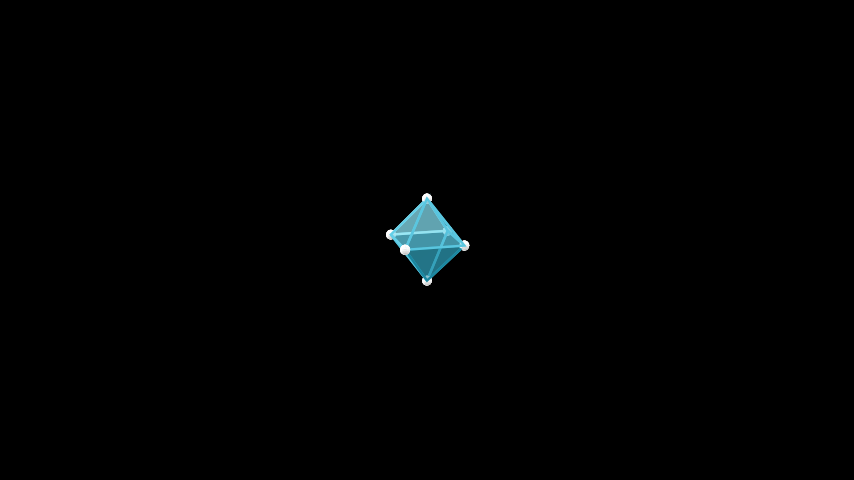# Octahedron¶

Qualified name: `manim.mobject.polyhedra.Octahedron`

class Octahedron(edge_length=1, **kwargs)[source]

An octahedron, one of the five platonic solids. It has 8 faces, 12 edges and 6 vertices.

Parameters

edge_length (float) – The length of an edge between any two vertices.

Examples

Example: OctahedronScene```from manim import *

class OctahedronScene(ThreeDScene):
def construct(self):
self.set_camera_orientation(phi=75 * DEGREES, theta=30 * DEGREES)
obj = Octahedron()
 `animate` Used to animate the application of a method. `animation_overrides` `depth` The depth of the mobject. `height` The height of the mobject. `width` The width of the mobject.